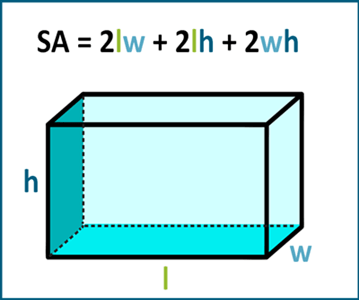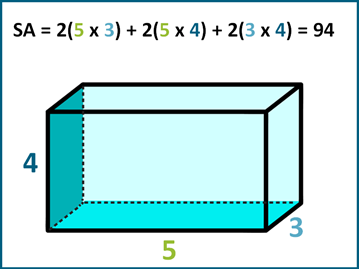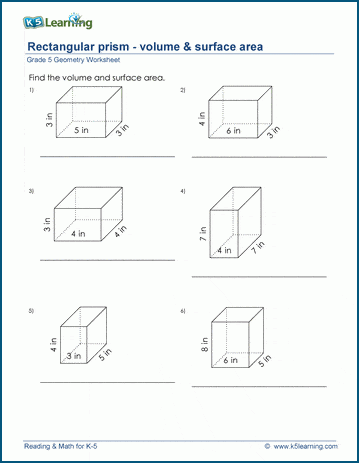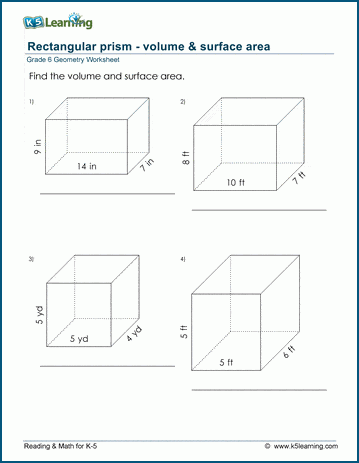# How to Find the Surface Area of Rectangular Prisms

## Surface area formula

The surface area of a rectangular prism is given by the formula:

SA = 2lw + 2lh + 2wh

where SA is the surface area, l is the length, w is the width, and h is the height.## How to calculate the surface area of a rectangular prism

Here's an example:

Suppose we have a rectangular prism with the following dimensions:

Length (l) = 5 cm

Width (w) = 3 cm

Height (h) = 4 cm

To find the surface area, we use the formula:

SA = 2lw + 2lh + 2wh =

2(5 cm x 3 cm) + 2(5 cm x 4 cm) + 2(3 cm x 4 cm) =

30 + 40 + 24 = 94 square cm

Therefore, the surface area of the rectangular prism is 94 square cm.Note that the units for surface area are square units (e.g. square cm, square inches, etc.).

## Grade 5 volume and surface area of rectangular prisms worksheets

In our worksheets, we combine the practice of calculating the volume and surface area of rectangular prisms. Here’s the set for grade 5.## Volume and surface area of rectangular prisms practice for grade 6

This is the set of worksheets for grade 6 for finding the volume and surface areas of rectangular prisms.Become a Member

This content is available to members only.